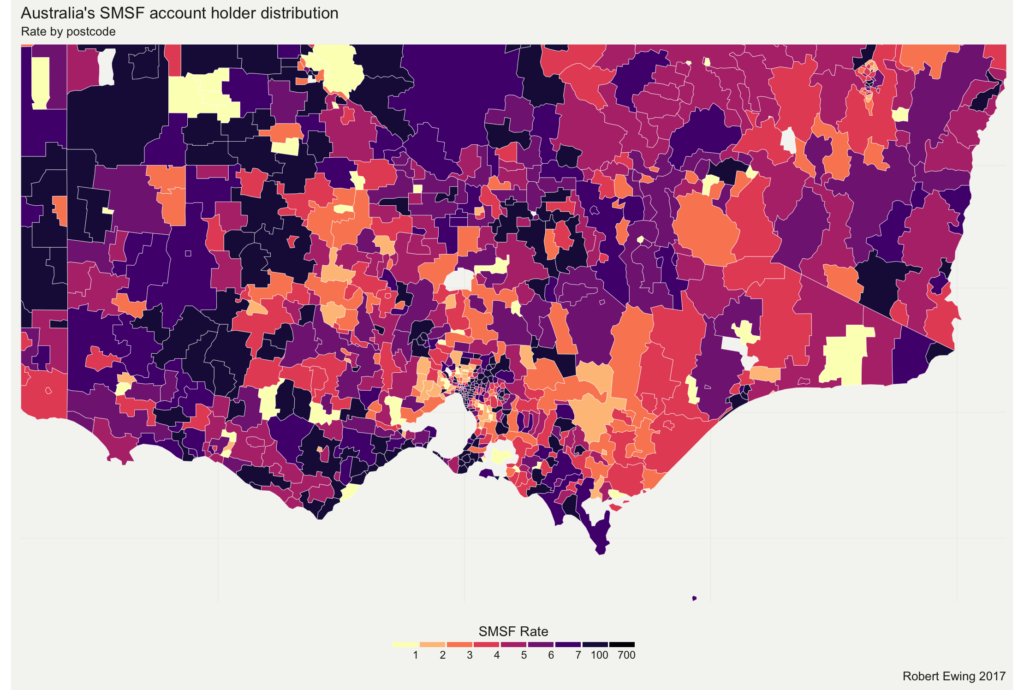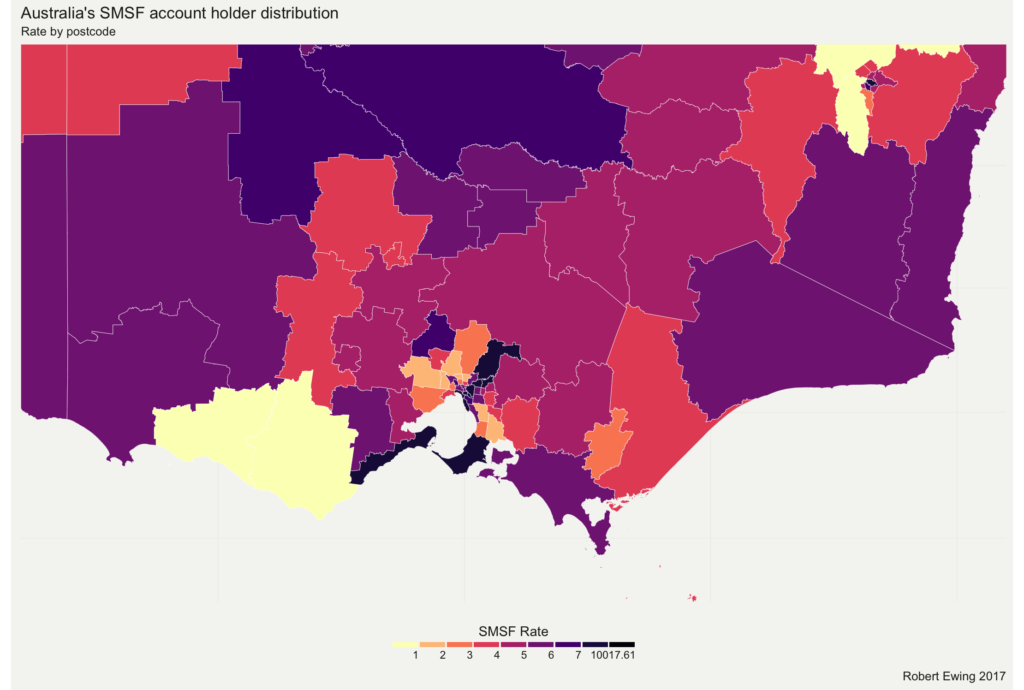## Pretty Mapping of Australia using R

January 5th, 2017

I’ve recently been experimenting with Data Visualisation in R. As part of that I’ve put together a little bit of (probably error ridden and redundant) code to help mapping of Australia.

First, my code is built on a foundation from Luke’s guide to building maps of Australia in R, and this guide to making pretty maps in R.

The problem is that a lot of datasets, particularly administrative ones, come with postcode as the only geographic information. And postcodes aren’t a very useful geographic structure – there’s no defined aggregation structure, they’re inconsistent in size, and heavily dependent on history.

For instance, a postcode level map of Australia looks like this:Way too messy to be useful.

The ABS has a nice set of statistical geography that will let me fix this problem by changing the aggregation level, but first I need to convert the data into another file.

Again, fortunately the ABS publishes concordances between postcodes and the Statistical Geography, so all I need to do is take those concordances and use them to mangle my data lightly. First, I used those concordances to make some CSV input files:

Concordance from Postcode to Statistical Area 2 level (2011)

Concordance from SA2 (2011) to SA2(2016)

Statistical Geography hierarchy to convert to SA3 and SA4

Then a little R coding. First convert from Postcode to SA2 (2011). SA level 2 is around the same level of detail of postcodes, and so the conversions won’t lose a lot of accuracy.And then convert to 2016 and add the rest of the geography:

`## Convert Postcode level data to ABS Statistical Geography heirarchy`

`## Quick hack job, January 2017`

`## Robert Ewing`

`require(dplyr)`

`## Read in original data file, clean as needed.`

`## This data file is expected to have a variable 'post' for the postcode,`

`## and a data series called 'smsf' for the numbers.`

`data_PCODE <- read.csv("SMSF2.csv", stringsAsFactors = FALSE)`

`## Change this line depending on your data series.`

`## This code is designed to read in only one series. If you need more than one,`

`## you'll need to change the Aggregate functions.`

`## Change this line to reflect the name of the data series in your file`

`data_PCODE\$x <- as.numeric(data_PCODE\$smsf)`

`data_PCODE\$smsf[is.na(data_PCODE\$x)] <- 0`

`data_PCODE\$POA_CODE16 <- sprintf("%04d", data_PCODE\$post)`

`## Read in concordance from Postcode to SA2 (2011)`

`concordance <- read.csv("PCODE_SA2.csv", stringsAsFactors = FALSE)`

`concordance\$POA_CODE16 <- sprintf("%04d", concordance\$POSTCODE)`

`## Join the files`

`working_data <- concordance %>% left_join(data_PCODE)`

`working_data\$x[is.na(working_data\$x)] <- 0`

`## Adjust for partial coverage ratios`

`working_data\$x_adj = working_data\$x * working_data\$Ratio`

`## And produce the SA2_2011 version of the dataset. Data is in x.`

`data_SA2_2011 <- aggregate(working_data\$x_adj,list(SA2_MAINCODE_2011 = working_data\$SA2_MAINCODE_2011),sum)`

`## Now read in the concordance from SA2_2011 to SA2_2016`

`concordance <- read.csv("SA2_2011_2016.csv", stringsAsFactors = FALSE)`

`## Join it.`

`working_data <- concordance %>% left_join(data_SA2_2011)`

`working_data\$x[is.na(working_data\$x)] <- 0`

`## Adjust for partial coverage ratios`

`working_data\$x_adj = working_data\$x * working_data\$Ratio`

`## And produce aggregate in SA2_2016`

`data_SA2_2016 <- aggregate(working_data\$x_adj,list(SA2_MAINCODE_2016 = working_data\$SA2_MAINCODE_2016),sum)`

`## and finally join the SA2 with the rest of the hierarchy to allow on the fly adjustment.`

`statgeo <- read.csv("SA2_3_4.csv", stringsAsFactors = FALSE)`

`data_SA2_2016 <- data_SA2_2016 %>% left_join(statgeo)`

The end result gives you a data set that can be converted to a higher level. Here's the chart above, but this time using SA3 rather than postcodes: